# Computer Network and Network Topology

### LESSON – COMPUTER NETWORK

###### TOPIC
• Introduction
• Types of Computer Network
• Computer Network Requirements
• Uses of Computer Network
• Network Topology
• Types of Network Topology
• Lesson Evaluation

###### INTRODUCTION

Computer network is the sharing of data (information) between a set of connected computers.

Computer networking has become one of the most successful ways of sharing information, where all computers are wirelessly linked together by a common network.

###### TYPES OF COMPUTER NETWORK

The types of computer network are as follows:

1. Local Area Network (LAN)

2. Metropolitan Area Network (MAN)

3. Wide Area Network (WAN)

1. LOCAL AREA NETWORK (LAN)

LAN is a set of computers connected together, usually within the same building.

For example – resident, school, laboratory, office within the same building.

2. METROPOLITAN AREA NETWORK (MAN)

MAN is a set of computers connected together, usually within the same city.

For example – Lagos state.

3. WIDE AREA NETWORK (WAN)

WAN is a world wide network.

It connects large number of computers together.

###### COMPUTER NETWORK REQUIREMENTS

The requirements for computer network are as follows:

1. Connecting cables

2. A central computer

3. Other computers

4. Switch or Hub

5. Network card for each computer

Main benefits of networks include:

1. It enhances communication and availability of information.

2. It allows for more convenient resource sharing.

For example, printers, scanners and copiers, or sharing software between multiple users, saves money.

3. It makes file sharing easier between different users.

4. It is highly flexible.

5. Sharing a single internet connection is cost-efficient and can help protect your systems if you properly secure the network. It is an inexpensive system.

6. It boosts and increase storage capacity such as images and music.

7. It increases cost efficiency.

The disadvantages of computer network are as follows:

1. It lacks privacy.

2. It poses security difficulties.

3. It lacks robustness.

4. It allows for more presence of computer viruses and malware.

5. Its light policing usage promotes negative acts.

6. It requires an expensive set-up.

###### USES OF COMPUTER NETWORK

The computer networks are useful to the organizations in the following ways:

1. Resource sharing.

2. For providing high reliability.

3. To save money.

4. It can provide a powerful communication medium.

###### MEANING OF NETWORK TOPOLOGY

Network topology is the arrangement and description of a computer network.

###### TYPES OF NETWORK TOPOLOGY

The types of computer network are as follows:

1. Bus topology

2. Ring topology

3. Star topology

1. BUS TOPOLOGY

Bus topology is a type of network where all the computers are connected to a single cable.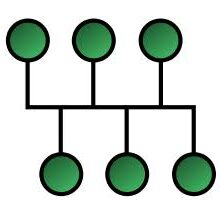2. RING TOPOLOGY

Ring topology is a type of network where  all computer are connected to create a circular data path.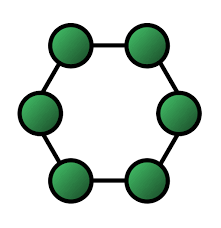3. STAR TOPOLOGY

Star topology is a network where all computer are connected at a central point.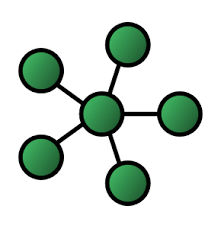3. MESH TOPOLOGY

Mesh topology is a network where all the computer are interconnected with one another.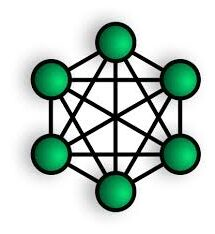5. TREE TOPOLOGY

Tree topology is a network where all the computers are connected like a branches of a tree.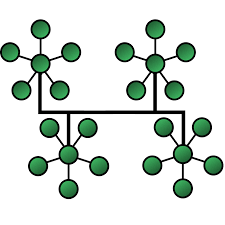6. POINT TO POINT TOPOLOGY

Point to point topology is a network where two or more computer are connected to one another direct in a line.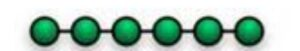###### LESSON EVALUATION

SECTION A

1. _______ is the sharing of data between computers.

A. Local Area Network

B. Large Area Network

C. Metropolitan Area Network

D. Computer Network

2. _______ is a set of computers connected together, usually within the same building.

A. Local Area Network

B. Large Area Network

C. Metropolitan Area Network

D. Computer Network

3. _______ is a set of computers connected together, usually within the same city.

A. Local Area Network

B. Large Area Network

C. Metropolitan Area Network

D. Computer Network

4. _______ is a world wide network.

A. Local Area Network

B. Large Area Network

C. Metropolitan Area Network

D. Computer Network

5. _______ connects large number of computers together.

A. Local Area Network

B. Large Area Network

C. Metropolitan Area Network

D. Computer Network

6. _______ is the arrangement and description of a computer network.

A. Computer network

B. Computer system

C. Computer network topology

D. Computer hardware

7. There are _______ types of network topology.

A. 3

B. 4

C. 5

D. 6

8. _______ types of network topology looks like a circle.

A. Bus topology

B. Ring topology

C. Star topology

D. Circle topology

9. _______ types of network topology looks like a sitting bus.

A. Bus topology

B. Ring topology

C. Star topology

D. Mesh topology

10. _______ types of network topology looks like a star.

A. Bus topology

B. Ring topology

C. Star topology

D. Circle topology

11. Network topology is the _______ and _______ of a computer network.

12. _______ is a type of network where all the computers are connected to a single cable.

A. Point to point topology

B. Ring topology

C. Bus topology

D. Mesh topology

13. _______ is a type of network where  all computer are connected to create a circular data path.

A. Bus topology

B. Ring topology

C. Star topology

D. Mesh topology

14. _______ is a network where all computer are connected at a central point.

A. Bus topology

B. Star topology

C. Tree topology

D. Ring topology

15. _______ is a network where all the computer are interconnected with one another.

A. Mesh topology

B. Ring topology

C. Star topology

D. Bus topology

16. _______ is a network where all the computers are connected like a branches of a tree.

A. Point to point topology

B. Ring topology

C. Tree topology

D. Mesh topology

17. _______ is a network where two or more computer are connected to one another direct in a line.

A. Point to point topology

B. Ring topology

C. Bus topology

D. Mesh topology

18. Bus topology is a network where two or more computer are connected to one another direct in a line.

A. True

B. False

19 Star topology is a network where all the computers are connected like a branches of a tree.

A. False

B. True

20. Mesh topology is a network where all the computer are interconnected with one another.

A. False

B. True

21. Bus topology is a type of network where all the computers are connected to a single cable.

A. False

B. True

SECTION B

1. explain the meaning of computer network.

2. state 3 types of computer network.

3. Write the full meaning of:

• LAN
• MAN
• WAN

4. define the following:

• LAN
• MAN
• WAN

5. list all the basic requirements for connecting two or more computers together.

6. state 4 advantages of computer network networking.

7. state 4 disadvantages of computer network networking.

8. State two uses of computer network.

9. explain the meaning of network topology.

10. list and explain any 5 network topology with appropriate drawing.

11. Sketch a mesh topology.

12. Sketch a star topology.

13. Sketch a bus topology.

14. Sketch a tree topology.